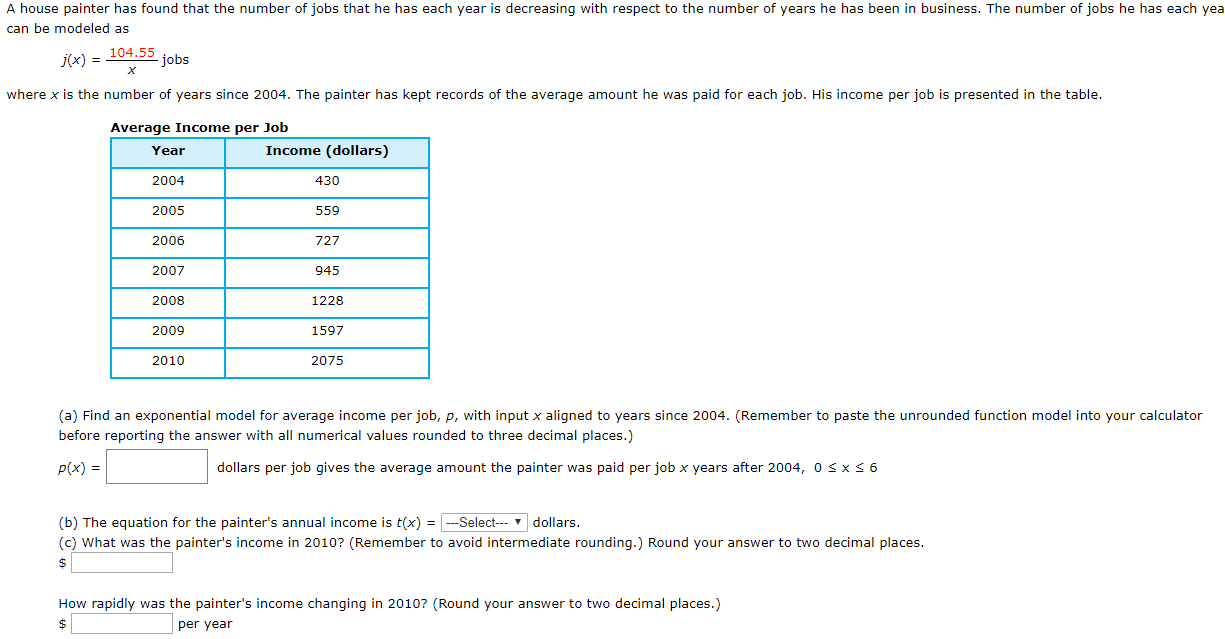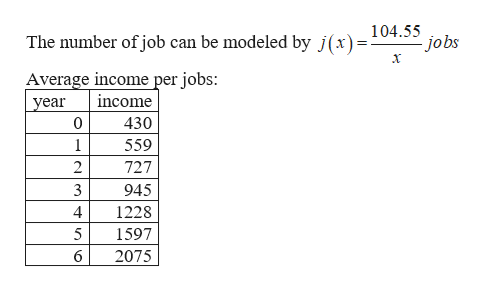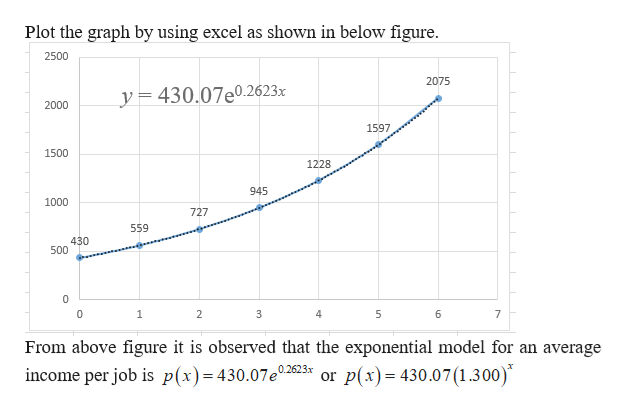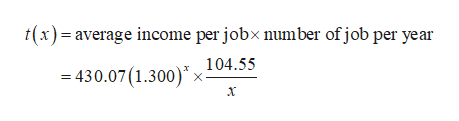# A house painter has found that the number of jobs that he has each year is decreasing with respect to the number of years he has been in business. The number of jobs he has each yeacan be modeled as104.55jobsXj(x) =where x is the number of years since 2004. The painter has kept records of the average amount he was paid for each job. His income per job is presented in the tableAverage Income per JobIncome (dollars)Year2004430200555920067272007945200812282009159720752010(a) Find an exponential model for average income per job, p, with input x aligned to years since 2004. (Remember to paste the unrounded function model into your calculatorbefore reporting the answer with all numerical values rounded to three decimal places.)p(x)dollars per job gives the average amount the painter was paid perjob x years after 2004, 0 s x 6(b) The equation for the painter's annual income is t(x) -Select dollars.(c) What was the painter's income in 2010? (Remember to avoid intermediate rounding.) Round your answer to two decimal places\$How rapidly was the painter's income changing in 2010? (Round your answer to two decimal places.)\$per year

Question
1 viewshelp_outlineImage TranscriptioncloseA house painter has found that the number of jobs that he has each year is decreasing with respect to the number of years he has been in business. The number of jobs he has each yea can be modeled as 104.55 jobs X j(x) = where x is the number of years since 2004. The painter has kept records of the average amount he was paid for each job. His income per job is presented in the table Average Income per Job Income (dollars) Year 2004 430 2005 559 2006 727 2007 945 2008 1228 2009 1597 2075 2010 (a) Find an exponential model for average income per job, p, with input x aligned to years since 2004. (Remember to paste the unrounded function model into your calculator before reporting the answer with all numerical values rounded to three decimal places.) p(x) dollars per job gives the average amount the painter was paid perjob x years after 2004, 0 s x 6 (b) The equation for the painter's annual income is t(x) -Select dollars. (c) What was the painter's income in 2010? (Remember to avoid intermediate rounding.) Round your answer to two decimal places \$ How rapidly was the painter's income changing in 2010? (Round your answer to two decimal places.) \$ per year fullscreen
check_circle

star
star
star
star
star
1 Rating
Step 1

(a)

given:help_outlineImage Transcriptionclose104.55 jobs The number of job can be modeled by j(x)= х Average income per jobs: income year 0 430 1 559 2 727 3 945 4 1228 5 1597 6 2075 fullscreen
Step 2

Find the exponential model for an average income per jobs as follows.help_outlineImage TranscriptionclosePlot the graph by using excel as shown in below figure 2500 2075 y= 430.07e0.2623x 2000 1597 1500 1228 945 1000 727 559 430 500 0 1 2 4 5 6 7 From above figure it is observed that the exponential model for an average or p(x) 430.07 1.300) income per job is p(x)= 430.07e02623x fullscreen
Step 3

(b)

Find the equation for the painter&rsq...help_outlineImage Transcriptioncloset(x)=average income per jobx number of job per year 104.55 430.07 (1.300) х X х fullscreen

### Want to see the full answer?

See Solution

#### Want to see this answer and more?

Solutions are written by subject experts who are available 24/7. Questions are typically answered within 1 hour.*

See Solution
*Response times may vary by subject and question.
Tagged in

### Other